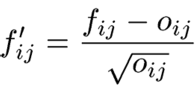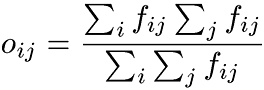Next:The Paradigmatic ApproachUp:Constructing a Domain Model Previous:Constructing a Domain Model

## The Syntagmatic Approach

The purpose of syntagmatic analysis is to find the strongest joints between sequential terms found in the corpus. Frequencies of different terms affect the probabilities of certain two terms next to each other. The strength of a joint between two successive terms is estimated by comparing the actual frequence of the co-occurence of the pair and its expected frequency.

The similarity rates$f$ij' of the terms i and j are calculated using the formulas:where$f$ij is the actual frequency of the pair ij, and $o$ij is its expected frequency.

If the actual frequency is considerably greater than expected, the joint is considered to be strong. This could be presented with a rule in which a nonterminal symbol produces the pair of sequential terms (e.g.$U$->ij).

In such a case, it can be further analyzed, e.g. using paradigmatic analysis, whether the distribution of a term pair is similar to the distribution of one of its members outside the pair.Next:The Paradigmatic ApproachUp:Constructing a Domain Model Previous:Constructing a Domain Model
Päivikki Parpola

Sat Oct 14 22:52:14 EEST 2000